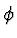CSE 5311 Fall 1999

Quiz #11

1.
(17 points.) Consider an RSA encryption of message M=100 with a public key pair = If p = 11, q = 7, and e = 7, what are the public and secret key pairs? what is the encryption of message M? If you cannot complete the calculations, clearly describe the process.

The value ofis (11 - 1) * (7 - 1) = 10 * 6 = 60. Applying ExtendedEuclid to e and, we get a value of d = 43. Thus the public key pair is (7, 77) and the secret key pair is (43, 77). The encrypted message can be obtained by applying ModularExponentiation to M = 100, e = 7, and n = 77, obtaining the ciphertext C = 23.

2.
(3 points.) What is an advantage of Public-Key Cryptography over Symmetric Cryptography?

Keys do not need to be distributed - decryption is dependent on a secret key that only the decryptor needs to know.